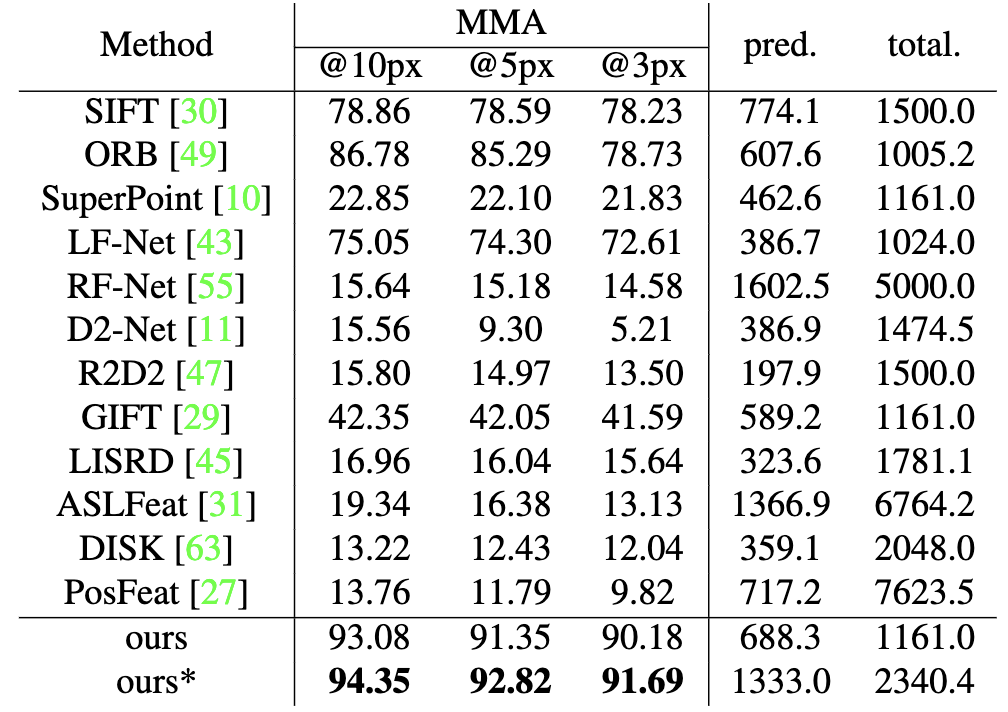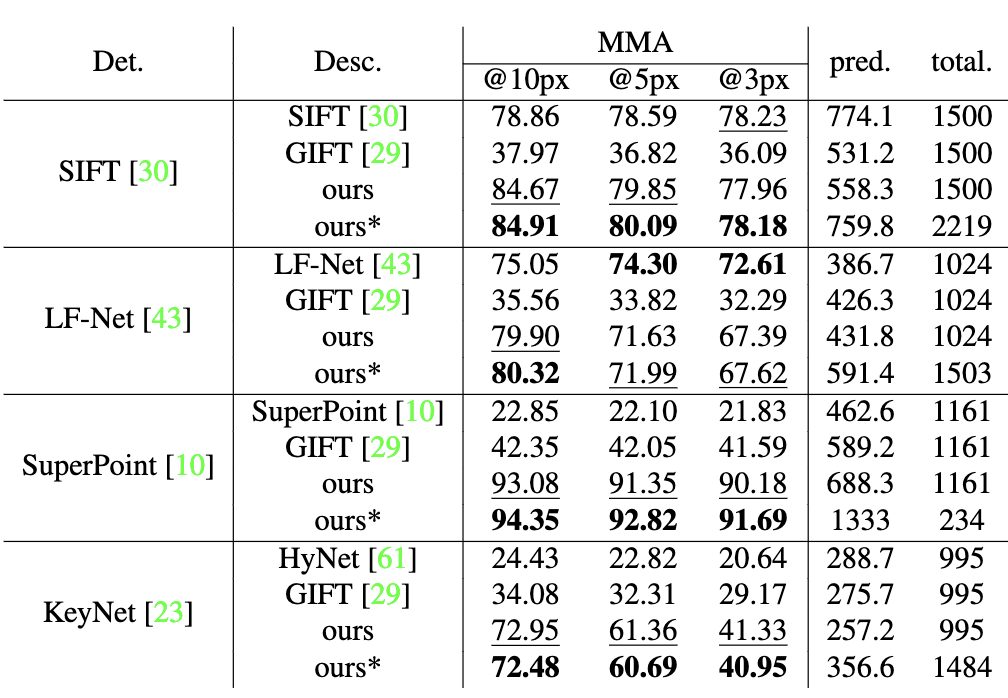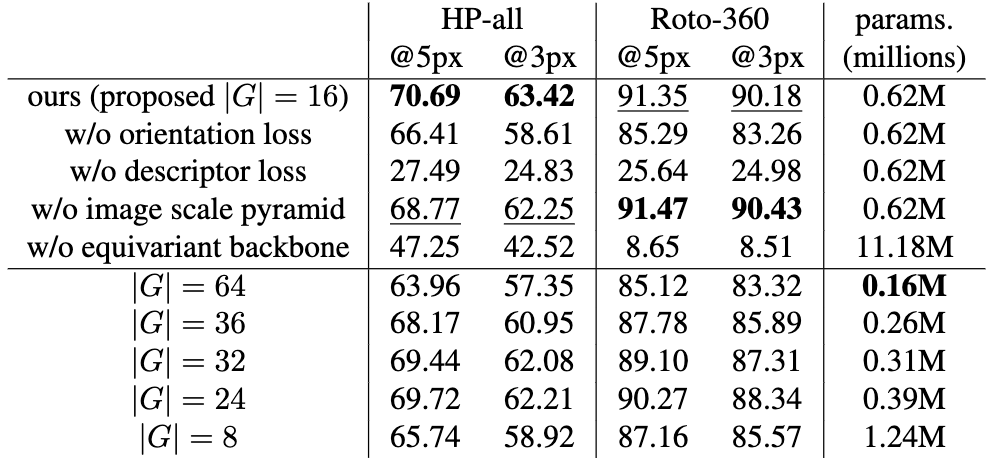## Abstract

Extracting discriminative local features that are invariant to imaging variations is an integral part of establishing correspondences between images. In this work, we introduce a self-supervised learning framework to extract discriminative rotation-invariant descriptors using group-equivariant CNNs. Thanks to employing group-equivariant CNNs, our method effectively learns to obtain rotation-equivariant features and their orientations explicitly, without having to perform sophisticated data augmentations. The resultant features and their orientations are further processed by group aligning, a novel invariant mapping technique that shifts the group-equivariant features by their orientations along the group dimension. Our group aligning technique achieves rotation-invariance without any collapse of the group dimension and thus eschews loss of discriminability. The proposed method is trained end-to-end in a self-supervised manner, where we use an orientation alignment loss for the orientation estimation and a contrastive descriptor loss for robust local descriptors to geometric/photometric variations. Our method demonstrates state-of-the-art matching accuracy among existing rotation-invariant descriptors under varying rotation and also show competitive results when transferred to the task of keypoint matching and camera pose estimation.

## Overview of Rotation-Equivariant Local Features (RELF)Figure 1. Overview of the proposed pipeline. An input image is forwarded through the equivariant networks to yield equivariant feature maps from multiple intermediate layers, encoding both low-level geometry and high-level semantic information. The feature maps are bilinearly interpolated to have equal spatial dimensions to be concatenated together. We use the first channel of the feature map F as the orientation histogram map O to predict the dominant orientations, which are used to shift the group-equivariant representation along the group dimension to yield discriminative rotation-invariant descriptors. To learn to extract accurate dominant orientation $\hat{\theta}$, we use the orientation alignment loss $\mathcal{L}^{\mathrm{ori}}$. To obtain descriptors robust to illumination and geometric changes, we use a contrastive descriptor loss $\mathcal{L}^{\mathrm{desc}}$ using the ground-truth homography $\mathcal{H}_{\mathrm{GT}}$.

## Group aligning vs. Group pooling / Learning dominant orientation of a keypoint(a) Difference between group pooling and group aligning. (b) Illustration of orientation alignment loss.

Figures 2 and 3. (a): In group pooling, the group dimension is collapsed to yield an invariant descriptor ($\mathbb{R}^{C\times |G|} \rightarrow \mathbb{R}^{C}$). In group aligning, the entire feature is cyclically shifted in the group dimension to obtain an invariant descriptor ($\mathbb{R}^{C\times |G|} \rightarrow \mathbb{R}^{C|G|}$) while preserving the group information and discriminability.
(b): Given two rotation-equivariant tensors $\textbf{p}^{\mathrm{A}}, \textbf{p}^{\mathrm{B}} \in \mathbb{R}^{C \times |G|}$ obtained from two different rotated versions of the same image, we apply cyclic shift on one of the descriptors in the group dimension using the GT difference in rotation. The orientation alignment loss supervises the output orientation vectors of the two descriptors to be the same.

## Comparison with existing invariant mapping operations(a) Evaluation with GT keypoint pairs on Roto-360 without training. (b) Evaluation with predicted keypoint pairs on Roto-360 with training.

Tables 1 and 2. (a) 'Align' uses GT rotation difference to apply group-aligning to demonstrate the upper-bound. 'None' does not use pooling nor aligning, demonstrating the lower-bound. We use an average of 111 keypoint pairs extracted using SuperPoint. The purpose is to compare the invariant mapping operations only while keeping the backbone network and the number of keypoints fixed.
(b) 'Max' and 'Avg' collapses the group dimension of the features through max pooling or average pooling. 'pred.' denotes the average number of predicted matches. We use an average of 1161 keypoint pairs extracted using SuperPoint. Overall, incorporating group aligning demonstrates the best results in terms of MMA compared to average pooling, max pooling or bilinear pooling in .

## Comparison with existing local descriptors on Roto-360(a) Comparison to existing local descriptors on Roto-360. (b) Comparison to existing local descriptors when using the same keypoint detector on Roto-360.

Tables 3 and 4. (a) We use mutual nearest matching for all methods to establish matches between images. 'total.' and 'pred.' denotes the average number of detected keypoints and predicted matches, respectively. 'ours*' denotes selecting multiple candidate descriptors based on the ratio of max value in the orientation histogram. We use SuperPoint keypoint detector same to the GIFT descriptor.
(b) Results in bold indicate the best result, and underlined results indicate the second best.

## Multiple descriptor extraction / Matching accuracy according to rotation degree(a) An example of multiple descriptor extraction. (b) Matching accuracies according to varying degree of rotations on Roto-360.

Figures 4 and 5. (a) The distribution is an orientation histogram $\textbf{o} \in \mathbb{R}^{16}$, and the scores are confidence values for each bin from group-equivariant features. Arrows indicate the orientation candidates for multiple descriptor extraction. The example shows selecting three orientations to obtain three candidate descriptors for a feature point, which is possible as we predict a score for each orientation.
(b) Our method shows the highest consistency, proving the enhanced invariance of descriptors obtained using group aligning against different rotations.

## Evaluation on HPatches / Ablation study(a) Evaluation with predicted keypoint pairs on HPatches. (b) Matching accuracies according to varying degree of rotations on Roto-360.

Tables 5 and 6. (a) The first group of methods includes existing local feature extraction methods. The second group of methods includes comparisons to other group pooling methods by replacing our group aligning with them. 'ours*' denotes the extraction of multiple descriptors using the orientation candidates, whose scores are at least 60\% of the maximum score in the orientation histogram. 'ours$\dagger$' denotes our method using the rotation-equivariant WideResNet16-8 (ReWRN) backbone for feature extraction. We use SuperPoint keypoint detector to evaluate ours.
(b) The second group of results shows the effect of the order of cyclic group $G$ on the performance of our method. 'params.' denotes the number of model parameters.

## Acknowledgements

This work was supported by Samsung Research Funding & Incubation Center of Samsung Electronics under Project Number SRFC-TF2103-02 and also by the NRF grant (NRF-2021R1A2C3012728) funded by the Korea government (MSIT).

## Paper

Learning Rotation-Equivariant Features for Visual Correspondence
Jongmin Lee, Byungjin Kim, Seungwook Kim, Minsu Cho
CVPR, 2023
[paper] [Bibtex]

## Code

Check our GitHub repository: [GitHub]

## References

•  Yuan Liu, Zehong Shen, Zhixuan Lin, Sida Peng, Hujun Bao, and Xiaowei Zhou. Gift: Learning transformation-invariant dense visual descriptors via group cnns. Advances in Neural Information Processing Systems, 32:6992–7003, 2019.